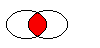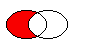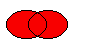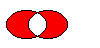# QRegion Class

The QRegion class specifies a clip region for a painter. More...

 Header: #include qmake: QT += gui

## Public Types

 enum RegionType { Rectangle, Ellipse } typedef const_iterator typedef const_reverse_iterator

## Public Functions

 QRegion() QRegion(int x, int y, int w, int h, RegionType t = Rectangle) QRegion(const QRect &r, RegionType t = Rectangle) QRegion(const QPolygon &a, Qt::FillRule fillRule = Qt::OddEvenFill) QRegion(const QRegion &r) QRegion(QRegion &&other) QRegion(const QBitmap &bm) const_iterator begin() const QRect boundingRect() const const_iterator cbegin() const const_iterator cend() const bool contains(const QPoint &p) const bool contains(const QRect &r) const const_reverse_iterator crbegin() const const_reverse_iterator crend() const const_iterator end() const QRegion intersected(const QRegion &r) const QRegion intersected(const QRect &rect) const bool intersects(const QRegion ®ion) const bool intersects(const QRect &rect) const bool isEmpty() const bool isNull() const const_reverse_iterator rbegin() const int rectCount() const QVector rects() const const_reverse_iterator rend() const void setRects(const QRect *rects, int number) QRegion subtracted(const QRegion &r) const void swap(QRegion &other) void translate(int dx, int dy) void translate(const QPoint &point) QRegion translated(int dx, int dy) const QRegion translated(const QPoint &p) const QRegion united(const QRegion &r) const QRegion united(const QRect &rect) const QRegion xored(const QRegion &r) const operator QVariant() const bool operator!=(const QRegion &other) const QRegion operator&(const QRegion &r) const QRegion operator&(const QRect &r) const QRegion & operator&=(const QRegion &r) QRegion & operator&=(const QRect &r) QRegion operator+(const QRegion &r) const QRegion operator+(const QRect &r) const QRegion & operator+=(const QRegion &r) QRegion & operator+=(const QRect &rect) QRegion operator-(const QRegion &r) const QRegion & operator-=(const QRegion &r) QRegion & operator=(const QRegion &r) QRegion & operator=(QRegion &&other) bool operator==(const QRegion &r) const QRegion operator^(const QRegion &r) const QRegion & operator^=(const QRegion &r) QRegion operator|(const QRegion &r) const QRegion & operator|=(const QRegion &r)
 QDataStream & operator<<(QDataStream &s, const QRegion &r) QDataStream & operator>>(QDataStream &s, QRegion &r)

## Detailed Description

The QRegion class specifies a clip region for a painter.

QRegion is used with QPainter::setClipRegion() to limit the paint area to what needs to be painted. There is also a QWidget::repaint() function that takes a QRegion parameter. QRegion is the best tool for minimizing the amount of screen area to be updated by a repaint.

This class is not suitable for constructing shapes for rendering, especially as outlines. Use QPainterPath to create paths and shapes for use with QPainter.

QRegion is an implicitly shared class.

### Creating and Using Regions

A region can be created from a rectangle, an ellipse, a polygon or a bitmap. Complex regions may be created by combining simple regions using united(), intersected(), subtracted(), or xored() (exclusive or). You can move a region using translate().

You can test whether a region isEmpty() or if it contains() a QPoint or QRect. The bounding rectangle can be found with boundingRect().

Iteration over the region (with begin(), end()) gives a decomposition of the region into rectangles. The same sequence of rectangles is returned by rects().

Example of using complex regions:

```void MyWidget::paintEvent(QPaintEvent *)
{
QRegion r1(QRect(100, 100, 200, 80),    // r1: elliptic region
QRegion::Ellipse);
QRegion r2(QRect(100, 120, 90, 30));    // r2: rectangular region
QRegion r3 = r1.intersected(r2);        // r3: intersection

QPainter painter(this);
painter.setClipRegion(r3);
...                                     // paint clipped graphics
}```

On Embedded Linux and X11 platforms, parts of this class rely on code obtained under the following licenses:

Permission is hereby granted, free of charge, to any person obtaining a copy of this software and associated documentation files (the "Software"), to deal in the Software without restriction, including without limitation the rights to use, copy, modify, merge, publish, distribute, sublicense, and/or sell copies of the Software, and to permit persons to whom the Software is furnished to do so, subject to the following conditions:

The above copyright notice and this permission notice shall be included in all copies or substantial portions of the Software.

THE SOFTWARE IS PROVIDED "AS IS", WITHOUT WARRANTY OF ANY KIND, EXPRESS OR IMPLIED, INCLUDING BUT NOT LIMITED TO THE WARRANTIES OF MERCHANTABILITY, FITNESS FOR A PARTICULAR PURPOSE AND NONINFRINGEMENT. IN NO EVENT SHALL THE X CONSORTIUM BE LIABLE FOR ANY CLAIM, DAMAGES OR OTHER LIABILITY, WHETHER IN AN ACTION OF CONTRACT, TORT OR OTHERWISE, ARISING FROM, OUT OF OR IN CONNECTION WITH THE SOFTWARE OR THE USE OR OTHER DEALINGS IN THE SOFTWARE.

Except as contained in this notice, the name of the X Consortium shall not be used in advertising or otherwise to promote the sale, use or other dealings in this Software without prior written authorization from the X Consortium.

Copyright 1987 by Digital Equipment Corporation, Maynard, Massachusetts.

Permission to use, copy, modify, and distribute this software and its documentation for any purpose and without fee is hereby granted, provided that the above copyright notice appear in all copies and that both that copyright notice and this permission notice appear in supporting documentation, and that the name of Digital not be used in advertising or publicity pertaining to distribution of the software without specific, written prior permission.

DIGITAL DISCLAIMS ALL WARRANTIES WITH REGARD TO THIS SOFTWARE, INCLUDING ALL IMPLIED WARRANTIES OF MERCHANTABILITY AND FITNESS, IN NO EVENT SHALL DIGITAL BE LIABLE FOR ANY SPECIAL, INDIRECT OR CONSEQUENTIAL DAMAGES OR ANY DAMAGES WHATSOEVER RESULTING FROM LOSS OF USE, DATA OR PROFITS, WHETHER IN AN ACTION OF CONTRACT, NEGLIGENCE OR OTHER TORTIOUS ACTION, ARISING OUT OF OR IN CONNECTION WITH THE USE OR PERFORMANCE OF THIS SOFTWARE.

## Member Type Documentation

### enum QRegion::RegionType

Specifies the shape of the region to be created.

ConstantValueDescription
`QRegion::Rectangle``0`the region covers the entire rectangle.
`QRegion::Ellipse``1`the region is an ellipse inside the rectangle.

### typedef QRegion::const_iterator

An iterator over the QRects that make up the region.

QRegion does not offer mutable iterators.

This typedef was introduced in Qt 5.8.

### typedef QRegion::const_reverse_iterator

A reverse iterator over the QRects that make up the region.

QRegion does not offer mutable iterators.

This typedef was introduced in Qt 5.8.

## Member Function Documentation

### QRegion::QRegion()

Constructs an empty region.

### QRegion::QRegion(intx, inty, intw, inth, RegionTypet = Rectangle)

Constructs a rectangular or elliptic region.

If t is `Rectangle`, the region is the filled rectangle (x, y, w, h). If t is `Ellipse`, the region is the filled ellipse with center at (x + w / 2, y + h / 2) and size (w ,h).

### QRegion::QRegion(const QRect &r, RegionTypet = Rectangle)

Create a region based on the rectange r with region type t.

If the rectangle is invalid a null region will be created.

### QRegion::QRegion(const QPolygon &a, Qt::FillRulefillRule = Qt::OddEvenFill)

Constructs a polygon region from the point array a with the fill rule specified by fillRule.

If fillRule is Qt::WindingFill, the polygon region is defined using the winding algorithm; if it is Qt::OddEvenFill, the odd-even fill algorithm is used.

Warning: This constructor can be used to create complex regions that will slow down painting when used.

### QRegion::QRegion(const QRegion &r)

Constructs a new region which is equal to region r.

### QRegion::QRegion(QRegion &&other)

Move-constructs a new region from region other. After the call, other is null.

This function was introduced in Qt 5.7.

### QRegion::QRegion(const QBitmap &bm)

Constructs a region from the bitmap bm.

The resulting region consists of the pixels in bitmap bm that are Qt::color1, as if each pixel was a 1 by 1 rectangle.

This constructor may create complex regions that will slow down painting when used. Note that drawing masked pixmaps can be done much faster using QPixmap::setMask().

### const_iterator QRegion::begin() const

Returns a const_iterator pointing to the beginning of the range of rectangles that make up this range, in the order in which rects() returns them.

This function was introduced in Qt 5.8.

### QRect QRegion::boundingRect() const

Returns the bounding rectangle of this region. An empty region gives a rectangle that is QRect::isNull().

### const_iterator QRegion::cbegin() const

Same as begin().

This function was introduced in Qt 5.8.

### const_iterator QRegion::cend() const

Same as end().

This function was introduced in Qt 5.8.

### bool QRegion::contains(const QPoint &p) const

Returns `true` if the region contains the point p; otherwise returns `false`.

### bool QRegion::contains(const QRect &r) const

Returns `true` if the region overlaps the rectangle r; otherwise returns `false`.

### const_reverse_iterator QRegion::crbegin() const

Same as rbegin().

This function was introduced in Qt 5.8.

### const_reverse_iterator QRegion::crend() const

Same as rend().

This function was introduced in Qt 5.8.

### const_iterator QRegion::end() const

Returns a const_iterator pointing to one past the end of the range of rectangles that make up this range, in the order in which rects() returns them.

This function was introduced in Qt 5.8.

### QRegion QRegion::intersected(const QRegion &r) const

Returns a region which is the intersection of this region and r.The figure shows the intersection of two elliptical regions.

This function was introduced in Qt 4.2.

### QRegion QRegion::intersected(const QRect &rect) const

Returns a region which is the intersection of this region and the given rect.

This function was introduced in Qt 4.4.

### bool QRegion::intersects(const QRegion &region) const

Returns `true` if this region intersects with region, otherwise returns `false`.

This function was introduced in Qt 4.2.

### bool QRegion::intersects(const QRect &rect) const

Returns `true` if this region intersects with rect, otherwise returns `false`.

This function was introduced in Qt 4.2.

### bool QRegion::isEmpty() const

Returns `true` if the region is empty; otherwise returns `false`. An empty region is a region that contains no points.

Example:

```QRegion r1(10, 10, 20, 20);
r1.isEmpty();               // false

QRegion r3;
r3.isEmpty();               // true

QRegion r2(40, 40, 20, 20);
r3 = r1.intersected(r2);    // r3: intersection of r1 and r2
r3.isEmpty();               // true

r3 = r1.united(r2);         // r3: union of r1 and r2
r3.isEmpty();               // false```

### bool QRegion::isNull() const

Returns `true` if the region is empty; otherwise returns `false`. An empty region is a region that contains no points. This function is the same as isEmpty

This function was introduced in Qt 5.0.

### const_reverse_iterator QRegion::rbegin() const

Returns a const_reverse_iterator pointing to the beginning of the range of rectangles that make up this range, in the reverse order in which rects() returns them.

This function was introduced in Qt 5.8.

### int QRegion::rectCount() const

Returns the number of rectangles that will be returned in rects().

This function was introduced in Qt 4.6.

### QVector<QRect> QRegion::rects() const

Returns an array of non-overlapping rectangles that make up the region.

The union of all the rectangles is equal to the original region.

### const_reverse_iterator QRegion::rend() const

Returns a const_reverse_iterator pointing to one past the end of the range of rectangles that make up this range, in the reverse order in which rects() returns them.

This function was introduced in Qt 5.8.

### void QRegion::setRects(const QRect *rects, intnumber)

Sets the region using the array of rectangles specified by rects and number. The rectangles must be optimally Y-X sorted and follow these restrictions:

• The rectangles must not intersect.
• All rectangles with a given top coordinate must have the same height.
• No two rectangles may abut horizontally (they should be combined into a single wider rectangle in that case).
• The rectangles must be sorted in ascending order, with Y as the major sort key and X as the minor sort key.

### QRegion QRegion::subtracted(const QRegion &r) const

Returns a region which is r subtracted from this region.The figure shows the result when the ellipse on the right is subtracted from the ellipse on the left (`left - right`).

This function was introduced in Qt 4.2.

### void QRegion::swap(QRegion &other)

Swaps region other with this region. This operation is very fast and never fails.

This function was introduced in Qt 4.8.

### void QRegion::translate(intdx, intdy)

Translates (moves) the region dx along the X axis and dy along the Y axis.

### void QRegion::translate(const QPoint &point)

Translates the region point.x() along the x axis and point.y() along the y axis, relative to the current position. Positive values move the region to the right and down.

Translates to the given point.

### QRegion QRegion::translated(intdx, intdy) const

Returns a copy of the region that is translated dx along the x axis and dy along the y axis, relative to the current position. Positive values move the region to the right and down.

This function was introduced in Qt 4.1.

### QRegion QRegion::translated(const QPoint &p) const

Returns a copy of the regtion that is translated p.x() along the x axis and p.y() along the y axis, relative to the current position. Positive values move the rectangle to the right and down.

This function was introduced in Qt 4.1.

### QRegion QRegion::united(const QRegion &r) const

Returns a region which is the union of this region and r.The figure shows the union of two elliptical regions.

This function was introduced in Qt 4.2.

### QRegion QRegion::united(const QRect &rect) const

Returns a region which is the union of this region and the given rect.

This function was introduced in Qt 4.4.

### QRegion QRegion::xored(const QRegion &r) const

Returns a region which is the exclusive or (XOR) of this region and r.The figure shows the exclusive or of two elliptical regions.

This function was introduced in Qt 4.2.

### QRegion::operator QVariant() const

Returns the region as a QVariant

### bool QRegion::operator!=(const QRegion &other) const

Returns `true` if this region is different from the other region; otherwise returns `false`.

### QRegion QRegion::operator&(const QRegion &r) const

Applies the intersected() function to this region and r. `r1&r2` is equivalent to `r1.intersected(r2)`.

### QRegion QRegion::operator&(const QRect &r) const

This function was introduced in Qt 4.4.

### QRegion &QRegion::operator&=(const QRegion &r)

Applies the intersected() function to this region and r and assigns the result to this region. `r1&=r2` is equivalent to `r1` = r1.intersected(r2).

### QRegion &QRegion::operator&=(const QRect &r)

This function was introduced in Qt 4.4.

### QRegion QRegion::operator+(const QRegion &r) const

Applies the united() function to this region and r. `r1+r2` is equivalent to `r1.united(r2)`.

### QRegion QRegion::operator+(const QRect &r) const

This function was introduced in Qt 4.4.

### QRegion &QRegion::operator+=(const QRegion &r)

Applies the united() function to this region and r and assigns the result to this region. `r1+=r2` is equivalent to `r1 = r1.united(r2)`.

### QRegion &QRegion::operator+=(const QRect &rect)

Returns a region that is the union of this region with the specified rect.

### QRegion QRegion::operator-(const QRegion &r) const

Applies the subtracted() function to this region and r. `r1-r2` is equivalent to `r1.subtracted(r2)`.

### QRegion &QRegion::operator-=(const QRegion &r)

Applies the subtracted() function to this region and r and assigns the result to this region. `r1-=r2` is equivalent to `r1 = r1.subtracted(r2)`.

### QRegion &QRegion::operator=(const QRegion &r)

Assigns r to this region and returns a reference to the region.

### QRegion &QRegion::operator=(QRegion &&other)

Move-assigns other to this QRegion instance.

This function was introduced in Qt 5.2.

### bool QRegion::operator==(const QRegion &r) const

Returns `true` if the region is equal to r; otherwise returns false.

### QRegion QRegion::operator^(const QRegion &r) const

Applies the xored() function to this region and r. `r1^r2` is equivalent to `r1.xored(r2)`.

### QRegion &QRegion::operator^=(const QRegion &r)

Applies the xored() function to this region and r and assigns the result to this region. `r1^=r2` is equivalent to `r1 = r1.xored(r2)`.

### QRegion QRegion::operator|(const QRegion &r) const

Applies the united() function to this region and r. `r1|r2` is equivalent to `r1.united(r2)`.

### QRegion &QRegion::operator|=(const QRegion &r)

Applies the united() function to this region and r and assigns the result to this region. `r1|=r2` is equivalent to `r1 = r1.united(r2)`.

## Related Non-Members

### QDataStream &operator<<(QDataStream &s, const QRegion &r)

Writes the region r to the stream s and returns a reference to the stream.